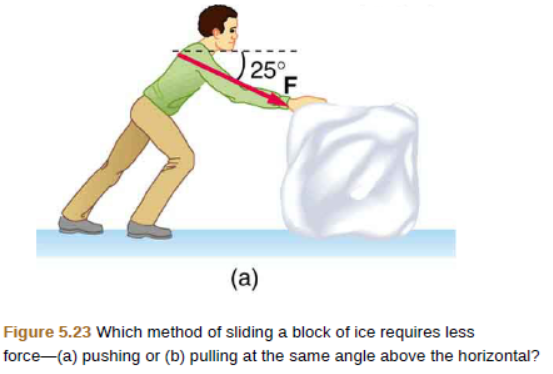# Problem: A contestant in a winter sporting event pushes a 45.0-kg block of ice across a frozen lake as shown in the figure. Gine the coefficient of static friction to be μs = 0.1, and the coefficient of kinetic friction to be μk = 0.03(a) Calculate the minimum force F he must exert to get the block moving. (b) What is the magnitude of its acceleration once it starts to move if that force is maintained?

###### FREE Expert Solution

(a)

In the y-direction:

N = mg + Fsin25°

In the x-direction:

Fcos 25° = μsN

Substitute for N in the equation in the x-direction.

81% (191 ratings)###### Problem Details

A contestant in a winter sporting event pushes a 45.0-kg block of ice across a frozen lake as shown in the figure. Gine the coefficient of static friction to be μ= 0.1, and the coefficient of kinetic friction to be μk = 0.03

(a) Calculate the minimum force F he must exert to get the block moving.

(b) What is the magnitude of its acceleration once it starts to move if that force is maintained?